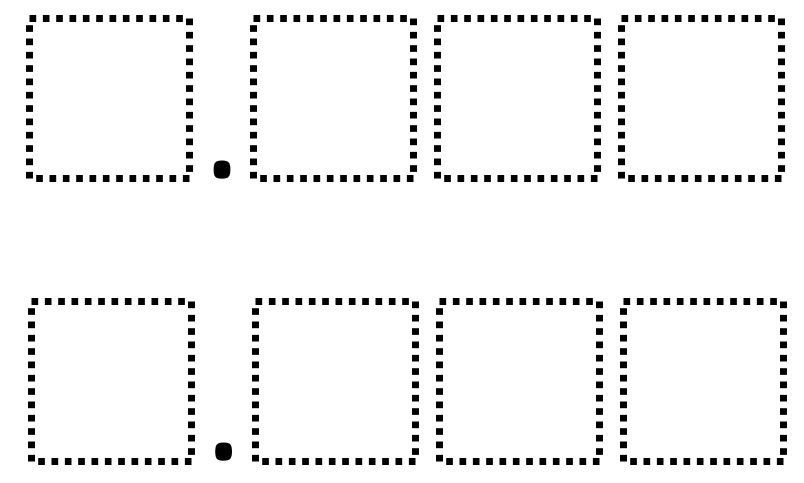# Rounding Decimals 2

Directions: Using the digits 0 to 9 at most one time each, place a digit in each box to create two different decimals that are equivalent when rounded to the nearest tenth.### Hint

How can you have values that are equivalent when rounded to the nearest tenth yet have different whole number values?

There are many possible answers including 5.971 and 6.043 which both round to 6.0.

Source: Robert Kaplinsky

## Equivalent Expressions with Fractions

Directions: Using the digits 0 to 9 at most one time each and choosing either …

1.2.